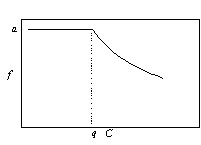### Orlando Sarnelle and Alan E. Wilson. 2008. Type III functional response in Daphnia. Ecology 89:1723–1732.

Appendix F. Fitting a Type I functional response to literature and experimental data.

We have taken two approaches to assess the fit of a Type I functional response. First, we asked whether there was a significant positive relationship between clearance rate and food concentration in our experimental data for food concentrations between 13 and 95 µg C/L (Fig. 4). The linear fit was highly significant (R2 = 0.31, P < 0.005) with a slope of 0.01 (SE = 0.003). Thus, the null (Type I) hypothesis of zero slope can be rejected, and a Type III functional response is statistically indicated by the positive slope. The value of the slope seems small but this is a function of the units of the axes. We discuss the magnitude of the change in clearance rate in the Discussion.

The second approach was to explicitly fit a Type I model to data from (1) the secondary analysis of mass-specific clearance rates (residuals for the no exhaustion data set in Table 2) and (2) the data from our experiment. This approach is somewhat problematic, because the Type I model with saturation is comprised of two functions joined by a conditional statement, and so must be fit by an iterative non-linear procedure (piecewise regression, see below). This precludes a rigorous statistical comparison between Type I and the other response types.

We fit the following model using the non-linear routine in JMP (SAS Institute):

 If C < q, f = a If C ≥ q, f = (aq)/C

where C is food concentration, f is clearance rate, q is the incipient limiting concentration (where f begins to decline with increasing C) and a is the maximum clearance rate. The function looks like this:We iteratively fit models with various combinations of values for a and q and chose the model with the lowest error mean square (MSe) as the best fitting model (JMP does not provide R2 estimates). We then compared the MSe of the best fitting Type I model to that of the corresponding polynomial fit. The MSe for the best fitting Type I models were 0.143 and 0.213 for the secondary analysis and the experiment, respectively. These values are higher than the MSE for the polynomial fits (0.096, 0.196, respectively), meaning that the Type I fit was poorer than the Type III fit in both cases.

LITERATURE CITED

JMP, The Statistical Discovery Software. 2003. SAS Institute Incorporated.

[Back to E089-103]